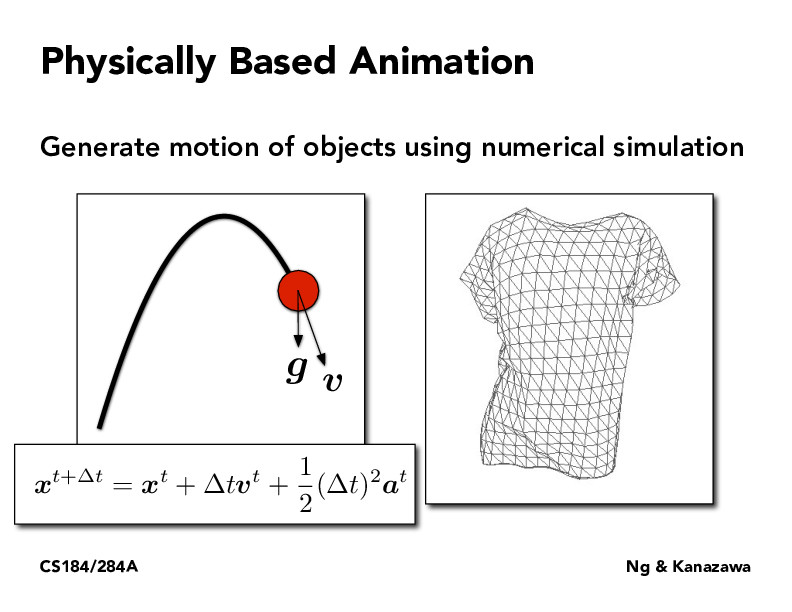Lecture 18: Introduction to Physical Simulation (2)philippe-eecs

Kinematic equation for 1D kinematics is shown. Though you have to split it up into x and y directions to understand how each velocity change. The y velocity will change since there is a force (gravity) acting on it, x velocity remains constant as there is no force.briana-jin-zhang

The equations here assume acceleration is constant, but I guess this is ok because it's for a certain time step t, which means even if there is change in acceleration, it is likely small.alexkassil

I think this is actually a 2d/3d kinematics formula, since all of x, v, and a are bolded, implying they are vectors that have their x/y (z) component

You must be enrolled in the course to comment